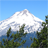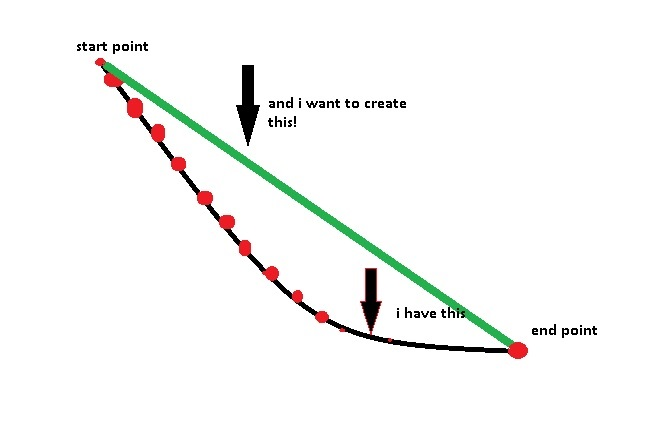# create line-chord from higher point to lower

4839
3
01-28-2015 07:04 AMOccasional Contributor III

1). Hello i want to create 2 species of line. The first i created it with straight segment form editing tools, as for the second i want it to have the same start and end point but to be drawn as a chord on the terrain, and not on the surface of it, to calculate the difference of their heights.Any help? Should i use traverse editing tool for that?

Tags (6)
1 Solution

Accepted SolutionsbyEsri Esteemed Contributor

You can use this code:

```import arcpy

fc_in = r"C:\Forum\test.gdb\Polyline_chord"
fc_out = r"C:\Forum\test.gdb\Polyline_straight"

sr = arcpy.Describe(fc_in).spatialReference

lines = []
with arcpy.da.SearchCursor(fc_in, ("SHAPE@")) as curs:
for row in curs:
polyline_in = row
polyline_out = arcpy.Polyline(arcpy.Array([polyline_in.firstPoint, polyline_in.lastPoint]), sr)
lines.append(polyline_out)

arcpy.CopyFeatures_management(lines, fc_out)```

Kind regards, Xander

3 RepliesbyEsri Esteemed Contributor

Can you explain what exactly it is you mean by "chord"? If you have a picture to explain what you are after that would be helpful too.Occasional Contributor IIIi want to create the green line which will be precisly above the black line, because i want to calculate the differences at their altitudes at the points with red colourbyEsri Esteemed Contributor

You can use this code:

```import arcpy

fc_in = r"C:\Forum\test.gdb\Polyline_chord"
fc_out = r"C:\Forum\test.gdb\Polyline_straight"

sr = arcpy.Describe(fc_in).spatialReference

lines = []
with arcpy.da.SearchCursor(fc_in, ("SHAPE@")) as curs:
for row in curs:
polyline_in = row
polyline_out = arcpy.Polyline(arcpy.Array([polyline_in.firstPoint, polyline_in.lastPoint]), sr)
lines.append(polyline_out)

arcpy.CopyFeatures_management(lines, fc_out)```

Kind regards, Xander